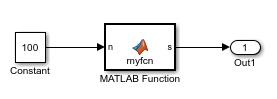## Use Dynamic Memory Allocation for Variable-Size Arrays in a MATLAB Function Block

This example shows how to use dynamic memory allocation for variable-size arrays in a MATLAB Function block. Dynamic memory allocation allocates memory on the heap as needed at run time, instead of allocating memory statically on the stack. Dynamic memory allocation is beneficial when:

• You do not know the upper bound of an array.

• You do not want to allocate memory on the stack for large arrays.

You can use dynamic memory allocation only for arrays that are local to the MATLAB Function block.

You cannot use dynamic memory allocation for:

• Input and output signals. Variable-size input and output signals must have an upper bound.

• Parameters or global variables. Parameters and global variables must be fixed-size.

• Fields of bus arrays. Bus arrays cannot have variable-size fields.

• Discrete state properties of System objects associated with a MATLAB System block.

### Create Model

Create this Simulink model that has a MATLAB Function block with an unbounded variable-size array.1. Create a Simulink® model `mymodel`.

2. Add a MATLAB Function block to the model.

3. In the MATLAB Function block, add this code:

```function s = myfcn(n) Z = rand(1,n); s = sum(Z); end```
4. Add a Constant block to the left of the MATLAB Function block.

5. Add an Outport block to the right of the MATLAB Function block.

6. Connect the blocks.

### Configure Model for Dynamic Memory Allocation

Make sure that you configure the model to use dynamic memory allocation for variable-size arrays in MATLAB Function blocks. In the Configuration Parameters dialog box, in the Simulation Target > Advanced parameters category, make sure that:

• The Dynamic memory allocation in MATLAB functions check box is selected.

• The Dynamic memory allocation threshold in MATLAB functions parameter has the default value 65536.

### Simulate Model Using Dynamic Memory Allocation

1. Simulate the model.

2. In the MATLAB Function Editor, to open the MATLAB® function report, click Function Report.

The variables tab shows that `Z` is a 1-by-:? array. The colon (:) indicates that the second dimension is variable size. The question mark (?) indicates that the second dimension is unbounded.

Simulation must use dynamic memory allocation for `Z` because the second dimension of `Z` does not have an upper bound.

### Use Dynamic Memory Allocation for Bounded Arrays

When an array is unbounded, the code generator must use dynamic memory allocation. If an array is bounded, the code generator uses dynamic memory allocation only if the array size, in bytes, is greater than or equal to the dynamic memory allocation threshold. The default value for this threshold is 65536.

Dynamic memory has a run-time performance cost. By controlling its use, you can improve execution speed.

If you make `Z` a bounded variable-size array with a size that is greater than the threshold, the code generator uses dynamic memory allocation for `Z`. For example:

1. In `mymodel`, modify `myfcn` so that `Z` has an upper bound of 500.

```function s = myfcn(n) assert(n < 500); Z = rand(1,n); s = sum(Z); end```

2. Simulate the model.

In the MATLAB function report, you see that `Z` is a 1-by-:500 array. It is variable size with an upper bound of 500.

3. Lower the dynamic memory allocation to a value less than or equal to `4000`, which is the size, in bytes, of `Z`. In the Configuration Parameters dialog box, in the Simulation Target > Advanced parameters category, set the Dynamic memory allocation threshold in MATLAB functions parameter to 4000.

4. Simulate the model.

The code generator uses dynamic memory allocation because the size of `Z` is equal to the dynamic memory allocation threshold, 4000.

### Generate C Code That Uses Dynamic Memory Allocation

If you have Simulink Coder™, you can generate C code for this model. Then, you can see how the code generator represents dynamically allocated arrays.

1. Configure the model to use a fixed-step solver. In the Configuration Parameters dialog box, in the Solver pane, under Solver selection:

• For Type, select `Fixed-step`.

• For Solver, select ```discrete (no continuous states)```.

2. Configure the model to create and use a code generation report. In the Configuration Parameters dialog box, in the Code Generation > Report pane, select Create code generation report and Open report automatically.

3. Edit the code in the MATLAB Function block so that it looks like this code:

```function s = myfcn(n) Z = rand(1,n); s = sum(Z); end```

`Z` is an unbounded variable-size array.

4. Make sure that the model is configured for dynamic memory allocation:

• The Dynamic memory allocation in MATLAB functions check box is selected.

• The Dynamic memory allocation threshold in MATLAB functions parameter has the default value 65536.

5. Build the model.

6. In the code generation report, open `mymodel.c`. You can tell that the code generator used dynamic memory allocation for `Z` because you see the `emxArray` type `emxArray_real_T_mymodel_T` and `emxArray` utility functions, such as `mymodel_emxInit_real_T`. The code generator uses an `emxArray` type for variables whose memory is dynamically allocated. The generated code uses the `emxArray` utility functions to manage the `emxArray`s.

If you have Embedded Coder®, you can customize the identifiers for `emxArray` types and the utility functions. See Identifier Format Control (Embedded Coder).